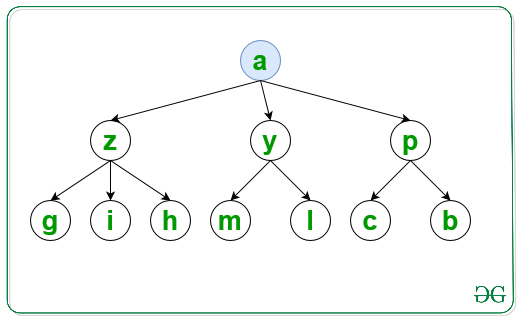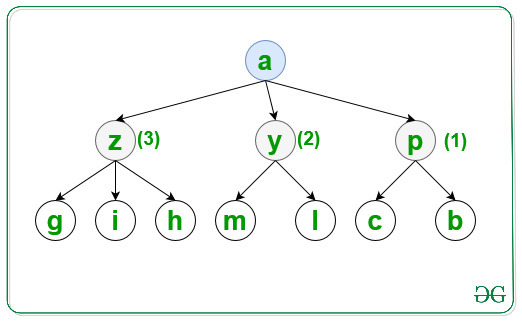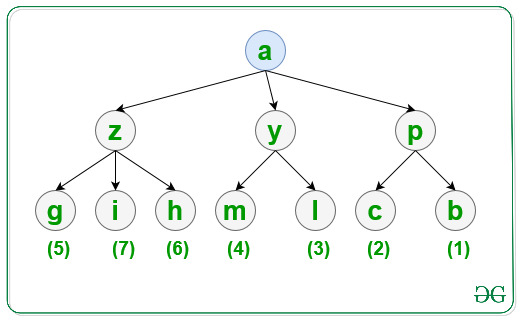# Traversal of a Graph in lexicographical order using BFS

• Last Updated : 20 Jul, 2021

Given a graph, G consisting of N nodes, a source S, and an array Edges[] of type {u, v} that denotes that there is an undirected edge between node u and v, the task is to traverse the graph in lexicographical order using BFS.

Input: N = 10, M = 10, S = ‘a’, Edges[] = { { ‘a’, ‘y’ }, { ‘a’, ‘z’ }, { ‘a’, ‘p’ }, { ‘p’, ‘c’ }, { ‘p’, ‘b’ }, { ‘y’, ‘m’ }, { ‘y’, ‘l’ }, { ‘z’, ‘h’ }, { ‘z’, ‘g’ }, { ‘z’, ‘i’ } }
Output: a p y z b c l m g h i

Attention reader! Don’t stop learning now. Get hold of all the important DSA concepts with the DSA Self Paced Course at a student-friendly price and become industry ready.  To complete your preparation from learning a language to DS Algo and many more,  please refer Complete Interview Preparation Course.

In case you wish to attend live classes with experts, please refer DSA Live Classes for Working Professionals and Competitive Programming Live for Students.

Explanation:
For the first level visit the node in lexicographical order as shown and print it:For the second level visit the node in lexicographical order as shown and print it:For the third level visit the node in lexicographical order as shown and print it:Input: N = 6, S = ‘a’, Edges[] = { { ‘a’, ‘e’ }, { ‘a’, ‘d’ }, { ‘e’, ‘b’ }, { ‘e’, ‘c’ }, { ‘d’, ‘f’ }, { ‘d’, ‘g’ } }
Output: a d e f g b c

Approach: Follow the steps below to solve the problem:

• Initialize a map, say G to store all the adjacent nodes of a node according to lexicographical order of the nodes.
• Initialize a map, say vis to check if a node is already traversed or not.
• Traverse the Edges[] array and store all the adjacent nodes of each node of the graph in G.
• Finally, traverse the graph using BFS and print the visited nodes of the graph.

Below is the implementation of the above approach:

## C++

 `// C++ program to implement``// the above approach` `#include ``using` `namespace` `std;` `// Function to traverse the graph in``// lexicographical order using BFS``void` `LexiBFS(map<``char``, set<``char``> >& G,``             ``char` `S, map<``char``, ``bool``>& vis)``{``    ``// Stores nodes of the graph``    ``// at each level``    ``queue<``char``> q;` `    ``// Insert nodes of first level``    ``q.push(S);` `    ``// Mark S as``    ``// visited node``    ``vis[S] = ``true``;` `    ``// Traverse all nodes of the graph``    ``while` `(!q.empty()) {` `        ``// Stores top node of queue``        ``char` `top = q.front();` `        ``// Print visited nodes of graph``        ``cout << top << ``" "``;` `        ``// Insert all adjacent nodes``        ``// of the graph into queue``        ``for` `(``auto` `i = G[top].begin();``             ``i != G[top].end(); i++) {` `            ``// If i is not visited``            ``if` `(!vis[*i]) {` `                ``// Mark i as visited node``                ``vis[*i] = ``true``;` `                ``// Insert i into queue``                ``q.push(*i);``            ``}``        ``}` `        ``// Pop top element of the queue``        ``q.pop();``    ``}``}` `// Utility Function to traverse graph``// in lexicographical order of nodes``void` `CreateGraph(``int` `N, ``int` `M, ``int` `S,``                 ``char` `Edges[])``{``    ``// Store all the adjacent nodes``    ``// of each node of a graph``    ``map<``char``, set<``char``> > G;` `    ``// Traverse Edges[] array``    ``for` `(``int` `i = 0; i < M; i++) {``        ``G[Edges[i]].insert(Edges[i]);``    ``}` `    ``// Check if a node is already visited or not``    ``map<``char``, ``bool``> vis;` `    ``LexiBFS(G, S, vis);``}` `// Driver Code``int` `main()``{``    ``int` `N = 10, M = 10 S = ``'a'``;``    ``char` `Edges[M]``        ``= { { ``'a'``, ``'y'` `}, { ``'a'``, ``'z'` `},``            ``{ ``'a'``, ``'p'` `}, { ``'p'``, ``'c'` `},``            ``{ ``'p'``, ``'b'` `}, { ``'y'``, ``'m'` `},``            ``{ ``'y'``, ``'l'` `}, { ``'z'``, ``'h'` `},``            ``{ ``'z'``, ``'g'` `}, { ``'z'``, ``'i'` `} };` `    ``// Function Call``    ``CreateGraph(N, M, S, Edges);` `    ``return` `0;``}`

## Java

 `// Java program to implement``// the above approach``import` `java.util.*;` `class` `Graph{` `// Function to traverse the graph in``// lexicographical order using BFS``static` `void` `LexiBFS(HashMap> G,``            ``char` `S, HashMap vis)``{``    ` `    ``// Stores nodes of the graph``    ``// at each level``    ``Queue q = ``new` `LinkedList<>();` `    ``// Insert nodes of first level``    ``q.add(S);` `    ``// Mark S as``    ``// visited node``    ``vis.put(S, ``true``);` `    ``// Traverse all nodes of the graph``    ``while` `(!q.isEmpty())``    ``{``        ` `        ``// Stores top node of queue``        ``char` `top = q.peek();` `        ``// Print visited nodes of graph``        ``System.out.print(top + ``" "``);` `        ``// Insert all adjacent nodes``        ``// of the graph into queue``        ``if` `(G.containsKey(top))``        ``{``            ``for``(``char`  `i : G.get(top))``            ``{``                ` `                ``// If i is not visited``                ``if` `(vis.containsKey(i))``                ``{``                    ``if` `(!vis.get(i))``                    ``{``                        ` `                        ``// Mark i as visited node``                        ``vis.put(i, ``true``);` `                        ``// Insert i into queue``                        ``q.add(i);``                    ``}``                ``}``                ``else``                ``{``                    ` `                    ``// Mark i as visited node``                    ``vis.put(i, ``true``);` `                    ``// Insert i into queue``                    ``q.add(i);``                ``}``            ``}``        ``}` `        ``// Pop top element of the queue``        ``q.remove();``    ``}``}` `// Utility Function to traverse graph``// in lexicographical order of nodes``static` `void` `CreateGraph(``int` `N, ``int` `M, ``char` `S,``                        ``char``[][] Edges)``{``    ` `    ``// Store all the adjacent nodes``    ``// of each node of a graph``    ``HashMap> G = ``new` `HashMap<>();` `    ``// Traverse Edges[] array``    ``for``(``int` `i = ``0``; i < M; i++)``    ``{``        ``if` `(G.containsKey(Edges[i][``0``]))``        ``{``            ``Set temp = G.get(Edges[i][``0``]);``            ``temp.add(Edges[i][``1``]);``            ``G.put(Edges[i][``0``], temp);``        ``}``        ``else``        ``{``            ``Set temp = ``new` `HashSet<>();``            ``temp.add(Edges[i][``1``]);``            ``G.put(Edges[i][``0``], temp);``        ``}``    ``}``    ` `    ``// Check if a node is already visited or not``    ``HashMap vis = ``new` `HashMap<>();` `    ``LexiBFS(G, S, vis);``}` `// Driver code``public` `static` `void` `main(String[] args)``{``    ``int` `N = ``10``, M = ``10``;``    ``char` `S = ``'a'``;``    ` `    ``char``[][] Edges = { { ``'a'``, ``'y'` `}, { ``'a'``, ``'z'` `},``                       ``{ ``'a'``, ``'p'` `}, { ``'p'``, ``'c'` `},``                       ``{ ``'p'``, ``'b'` `}, { ``'y'``, ``'m'` `},``                       ``{ ``'y'``, ``'l'` `}, { ``'z'``, ``'h'` `},``                       ``{ ``'z'``, ``'g'` `}, { ``'z'``, ``'i'` `} };` `    ``// Function Call``    ``CreateGraph(N, M, S, Edges);``}``}` `// This code is contributed by hritikrommie`

## Python3

 `# Python3 program to implement``# the above approach``from` `collections ``import` `deque` `G ``=` `[[] ``for` `i ``in` `range``(``1000``)]``vis ``=` `[``False` `for` `i ``in` `range``(``1000``)]` `# Function to traverse the graph in``# lexicographical order using BFS``def` `LexiBFS(S):``    ` `    ``global` `G, vis``    ` `    ``# Stores nodes of the graph``    ``# at each level``    ``q ``=` `deque()``    ` `    ``# Insert nodes of first level``    ``q.append(``ord``(S))` `    ``# Mark S as``    ``# visited node``    ``vis[``ord``(S)] ``=` `True``    ``#a = []` `    ``# Traverse all nodes of the graph``    ``while` `(``len``(q) > ``0``):``        ` `        ``# Stores top node of queue``        ``top ``=` `q.popleft()``        ``print``(``chr``(top), end ``=` `" "``)` `        ``# Insert all adjacent nodes``        ``# of the graph into queue``        ``for` `i ``in` `G[top]:``            ` `            ``# If i is not visited``            ``if` `(``not` `vis[i]):``                ``#print(chr(i),end=" ")` `                ``# Mark i as visited node``                ``vis[i] ``=` `True` `                ``# Insert i into queue``                ``q.append(i)` `# Utility Function to traverse graph``# in lexicographical order of nodes``def` `CreateGraph(N, M, S,Edges):` `    ``# Traverse Edges[] array``    ``for` `i ``in` `range``(M):``        ``G[``ord``(Edges[i][``0``])].append(``ord``(Edges[i][``1``]))` `    ``for` `i ``in` `range``(``1000``):``        ``G[i] ``=` `sorted``(G[i])` `    ``LexiBFS(S)` `# Driver Code``if` `__name__ ``=``=` `'__main__'``:``    ` `    ``N, M ``=` `10``, ``10``    ``S ``=` `'a'``    ` `    ``Edges ``=` `[ [ ``'a'``, ``'y'` `], [ ``'a'``, ``'z'` `],``              ``[ ``'a'``, ``'p'` `], [ ``'p'``, ``'c'` `],``              ``[ ``'p'``, ``'b'` `], [ ``'y'``, ``'m'` `],``              ``[ ``'y'``, ``'l'` `], [ ``'z'``, ``'h'` `],``              ``[ ``'z'``, ``'g'` `], [ ``'z'``, ``'i'` `] ]` `    ``# Function Call``    ``CreateGraph(N, M, S, Edges)` `# This code is contributed by mohit kumar 29`

## Javascript

 ``
Output:
`a p y z b c l m g h i`

Time Complexity: O(N * log(N))
Auxiliary Space: O(N)

My Personal Notes arrow_drop_up